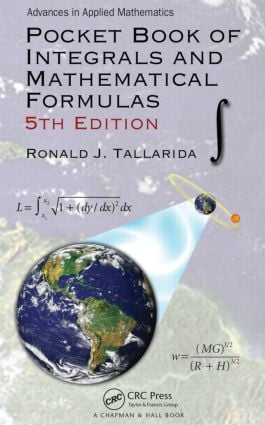# Pocket Book of Integrals and Mathematical Formulas

## 5th Edition

Chapman and Hall/CRC

387 pages | 61 B/W Illus.

##### Purchasing Options:\$ = USD
Paperback: 9781498704755
pub: 2015-03-20
\$33.95
x
Hardback: 9781138441668
pub: 2017-07-27
\$205.00
x
eBook (VitalSource) : 9780429160783
pub: 2015-03-20
from \$16.48

FREE Standard Shipping!

### Description

Pocket Book of Integrals and Mathematical Formulas, 5th Edition covers topics ranging from precalculus to vector analysis and from Fourier series to statistics, presenting numerous worked examples to demonstrate the application of the formulas and methods. This popular pocket book is an essential source for students of calculus and higher mathematics courses. It also provides an easy-to-use, accessible reference for mathematicians, engineers, scientists, and others seeking vital mathematical formulas, concepts, and definitions.

Enlarging the type without sacrificing special topics involving financial mathematics and number theory, this 5th Edition:

• Includes several classic calculus applications that illustrate the power and practical use of calculus
• Discusses an interesting offshoot of Fermat’s last theorem, namely, "near misses"
• Reformats and revises the table of integrals for improved clarity and accuracy

Through careful selection of topics and detail, Pocket Book of Integrals and Mathematical Formulas, 5th Edition remains a portable yet comprehensive resource for students and professionals, containing the most important mathematical formulas for engineering and scientific applications.

### Table of Contents

Preface to the Fifth Edition

Preface to the Fourth Edition

Preface to the Third Edition

Preface to the Second Edition

Preface to the First Edition

Author

Greek Letters

Elementary Algebra and Geometry

Fundamental Properties (Real Numbers)

Exponents

Fractional Exponents

Irrational Exponents

Logarithms

Factorials

Binomial Theorem

Factors and Expansion

Progression

Complex Numbers

Polar Form

Permutations

Combinations

Algebraic Equations

Geometry

Pythagorean Theorem

Determinants, Matrices, and Linear Systems of Equations

Determinants

Evaluation by Cofactors

Properties of Determinants

Matrices

Operations

Properties

Transpose

Identity Matrix

Adjoint

Inverse Matrix

Systems of Linear Equations

Matrix Solution

Trigonometry

Triangles

Trigonometric Functions of an Angle

Trigonometric Identities

Inverse Trigonometric Functions

Analytic Geometry

Rectangular Coordinates

Distance between Two Points: Slope

Equations of Straight Lines

Distance from a Point to a Line

Circle

Parabola

Ellipse

Hyperbola (e > 1)

Change of Axes

General Equation of Degree 2

Polar Coordinates

Curves and Equations

Exponential Function (Half-Life)

Series, Number Facts, and Theory

Bernoulli and Euler Numbers

Series of Functions

Error Function

Fermat’s Little Theorem

Fermat’s Last Theorem

Beatty’s Theorem

An Interesting Prime

Goldbach Conjecture

Twin Primes

Collatz Conjecture

Differential Calculus

Notation

Slope of a Curve

Angle of Intersection of Two Curves

Radius of Curvature

Relative Maxima and Minima

Points of Inflection of a Curve

Taylor’s Formula

Indeterminant Forms

Numerical Methods

Functions of Two Variables

Partial Derivatives

Application of Derivatives

Related Rate Problems

Rectilinear Motion

Applied Problem in Maximum and Minimum

Integral Calculus

Indefinite Integral

Definite Integral

Properties

Common Applications of the Definite Integral

Cylindrical and Spherical Coordinates

Double Integration

Surface Area and Volume by Double Integration

Centroid

Applications of Integration

Indefinite Integral

The Definite Integral

Vector Analysis

Vectors

Vector Differentiation

Divergence Theorem (Gauss)

Stokes’ Theorem

Planar Motion in Polar Coordinates

Geostationary Satellite Orbit

Special Functions

Hyperbolic Functions

Gamma Function (Generalized Factorial Function)

Laplace Transforms

z-Transform

Fourier Series

Functions with Period Other than 2π

Bessel Functions

Legendre Polynomials

Laguerre Polynomials

Hermite Polynomials

Orthogonality

Differential Equations

First-Order, First-Degree Equations

Second-Order Linear Equations (with Constant Coefficients)

Runge Kutta Method (of Order 4)

Statistics

Arithmetic Mean

Median

Mode

Geometric Mean

Harmonic Mean

Variance

Standard Deviation

Coefficient of Variation

Probability

Binomial Distribution

Mean of Binomially Distributed Variable

Normal Distribution

Poisson Distribution

Empirical Distributions

Estimation

Hypotheses Testing

t-Distribution

Hypothesis Testing with t- and Normal Distributions

Chi-Square Distribution

Least Squares Regression

Nonlinear Regression Analysis

The F-Distribution (Analysis of Variance)

Summary of Probability Distributions

Sample Size Determinations

Financial Mathematics

Simple Interest

True Interest Formula (Loan Payments)

Loan Payment Schedules

Loan Balance Calculation

Accelerated Loan Payment

Lump Sum Payment

Compound Interest

Time to Double (Your Money)

Present Value of a Single Future Payment

Regular Saving to Accumulate a Specified Amount

Monthly Payments to Achieve a Specified Amount

Periodic Withdrawals from an Interest-Bearing Account

Periodic Withdrawals That Maintain the Principal

Time to Deplete an Interest-Bearing Account with Periodic Withdrawals

Amounts to Withdraw for a Specified Number of Withdrawals I: Payments at the End of Each Year

Amounts to Withdraw for a Specified Number of Withdrawals II: Payments at the Beginning of Each Year

Present Value of Regular Payments

Annuities

The In-Out Formula

Stocks and Stock Quotations

Bonds

Tax-Free Yield

Stock Options (Puts and Calls)

Market Averages

Mutual and Quotations

Dollar Cost Averaging

Moving Average

Table of Derivatives

Table of Integrals: Indefinite and Definite Integrals

Appendix

Index

### About the Author

Ronald J. Tallarida holds a BS and MS in physics/mathematics and a Ph.D in pharmacology. His primary appointment is professor of pharmacology at Temple University School of Medicine, Philadelphia, Pennsylvania, USA. For more than 30 years, he also served as adjunct professor of biomedical engineering at Drexel University, Philadelphia, Pennsylvania, USA, where he received the Lindback Award for distinguished teaching of mathematics. He has published more than 290 works, been the recipient of NIH research grants, and served as consultant to industry and government agencies. His research interests include the mathematical modeling of biological systems, feedback control, and the action of drugs and drug combinations.

### Subject Categories

##### BISAC Subject Codes/Headings:
MAT003000
MATHEMATICS / Applied
MAT004000
MATHEMATICS / Arithmetic
MAT037000
MATHEMATICS / Functional Analysis